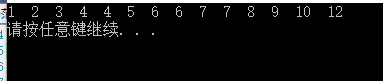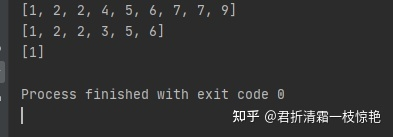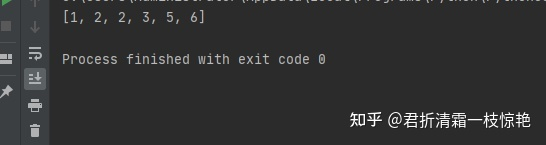• 题目连接：合并两个有序的数组 题目描述： 给出两个有序的整数数组 和 ，请将数组 合并到数组 中，变成一个有序的数组 注意： 可以假设数组有足够的空间存放 数组的元素， 和 中初始的元素数目分别为m和n 解法一：最...
题目连接：合并两个有序的数组
题目描述：
给出两个有序的整数数组 和 ，请将数组 合并到数组 中，变成一个有序的数组
注意：
可以假设数组有足够的空间存放 数组的元素， 和 中初始的元素数目分别为m和n
解法一：最简单解法，暴力求解（合并两数组+冒泡排序）

100.00% 通过率 4ms 运行时间 504KB 占用内存

#include <cstring>
class Solution {
public:
void merge(int A[], int m, int B[], int n) {
//合并数组
for(int index_a = m, index_B = 0; index_a < m+n; index_a++, index_B++)
A[index_a] = B[index_B];
for(int i = 0; i < m+n; i++)
for(int j = 0; j < m+n-1; j++)
{
if(A[j] > A[j+1])
{
int t = A[j];
A[j] = A[j+1];
A[j+1] = t;
}
}
}
}

解法二：从尾到头比较插入

100.00% 通过率 3ms 运行时间 480KB 占用内存

#include <cstring>
class Solution {
public:
void merge(int A[], int m, int B[], int n) {
//B数组为空直接返回
if(n == 0)
return ;
//A为空但是B不为空
else if(m == 0)
{
int index_AB = 0;
for(index_AB = 0; index_AB < n; index_AB++)
A[index_AB] = B[index_AB];
}
//正常情况
//从后往前放数据
else
{
int index = m+n-1;
int index_A = m-1;
int index_B = n-1;
while(index_A > -1 && index_B > -1)
{
if(A[index_A] > B[index_B])
{
A[index--] = A[index_A];
index_A--;
}
else
{
A[index--] = B[index_B];
index_B--;
}
}
if(index_A < 0)
while(index_B > -1)
{
A[index--] = B[index_B--];
}

}
}
};



展开全文• 合并两个有序的数组
环境：win10，vs2103 算法：
本题的题眼是”有序的数组“，我们可以利用两个有序的数组。

例如：给出两个增序数组

  先创建一个新数组，此数组的大小大于或者等于两个已知数组大小的和。通过比较两个有序数组中的元素，谁小就把谁放到空数组，直到其中一个数组为空，最后把剩下的数组全部放到创建的数组里

代码：

#include<iostream>
#include<stdio.h>
#include<stdlib.h>
using namespace std;
int* Merge(int*array1,int size1, int* array2,int size2)
{
int index1 = 0;
int index2 = 0;
int index = 0;
int*pTemp = new int(size1 + size2);//创建一个大小为已知两个数组大小和的数组，用pTemp标记
while (index1 < size1&&index2 < size2)//循环到其中一个数组为空，就退出循环
{
if (array1[index1] < array2[index2])//如果数组1的元素小于数组2的元素，就把数组1的元素放到新数组
pTemp[index++] = array1[index1++];
else
pTemp[index++] = array2[index2++];//否则就把数组2的元素放到新数组
}
if (array1[index1])//退出循环后，如果数组1的元素未空，就把数组1的元素都放到新数组中，因为数组1是有序的，所以不用排序
pTemp[index++] = array1[index1++];
if (array2[index2])//退出循环后，如果数组2的元素未空，就把数组2的元素都放到新数组中，因为数组2是有序的，所以不用排序
pTemp[index++] = array2[index2++];
return pTemp;
}
int main()
{
int *p;
int size1 = 0;
int size2 = 0;
//int num = 0;
int array1[] = { 1, 4, 6, 7, 9, 12 };
int array2[] = { 2, 3, 4, 5, 6, 7, 8, 10 };
size1 = sizeof(array1) /sizeof (array1);//数组1的元素个数
size2 = sizeof(array2) / sizeof (array2); //数组2的元素个数
int num = size1 + size2;
int array = { 0 };//新数组，此数组的大小必须大于或者等于数组1和数组2的大小之和
p=Merge(array1, size1, array2, size2);
for (int i = 0; i < num; ++i)//打印新数组
{
array[i] = *p++;
printf("%d  ", array[i]);
}
printf("\n");
system("pause");
return 0;
}

运行结果：展开全文• 88. 合并两个有序数组 题目给你两个有序整数数组 nums1 和 nums2，请你将 nums2 合并到 nums1 中，使 nums1 成为一个有序数组。 初始化 nums1 和 nums2 元素数量分别为 m 和 n 。你可以假设 nums1 有足够空间...
88. 合并两个有序数组 题目给你两个有序整数数组 nums1 和 nums2，请你将 nums2 合并到 nums1 中，使 nums1 成为一个有序数组。

初始化 nums1 和 nums2 的元素数量分别为 m 和 n 。你可以假设 nums1 有足够的空间（空间大小等于 m + n）来保存 nums2 中的元素。

示例 1：
输入：nums1 = [1,2,3,0,0,0], m = 3, nums2 = [2,5,6], n = 3
输出：[1,2,2,3,5,6]

示例 2：
输入：nums1 = , m = 1, nums2 = [], n = 0
输出：

代码：#合并两个数组
class Solution(object):
def merge(self, nums1, m, nums2, n):
"""
:type nums1: List[int]
:type m: int
:type nums2: List[int]
:type n: int
:rtype: void Do not return anything, modify nums1 in-place instead.
"""
nums1[:] = sorted(nums1[:m] + nums2)
return nums1

if __name__ == '__main__':
numA = [1, 2, 5, 7, 9]
numB = [2, 4, 6, 7]
print(Solution().merge(numA, 5, numB, 4))
print(Solution().merge([1,2,3,0,0,0], 3, [2,5,6], 3)) #[1,2,2,3,5,6]
print(Solution().merge(, 1, [], 0)) #运行：方法2：#88. 合并两个有序数组
#给你两个有序整数数组 nums1 和 nums2，请你将 nums2 合并到 nums1 中，使 nums1 成为一个有序数组。
# 初始化ums1 和 nums2 的元素数量分别为m 和 n 。
# 你可以假设nums1有足够的空间（空间大小大于或等于m + n）来保存 nums2 中的元素。

class Solution:
def mergeB(self, nums1, m, nums2, n):
# Make a copy of nums1.
nums1_copy = nums1[:m]
nums1[:] = []

# Two get pointers for nums1_copy and nums2.
p1 = 0
p2 = 0

# Compare elements from nums1_copy and nums2
# and add the smallest one into nums1.
while p1 < m and p2 < n:
if nums1_copy[p1] < nums2[p2]:
nums1.append(nums1_copy[p1])
p1 += 1
else:
nums1.append(nums2[p2])
p2 += 1

# if there are still elements to add
if p1 < m:
nums1[p1 + p2:] = nums1_copy[p1:]
if p2 < n:
nums1[p1 + p2:] = nums2[p2:]
return nums1

nums1 = [1, 2, 3, 0, 0, 0]
nums2 = [2, 5, 6]
n1 = Solution()
print(n1.mergeB(nums1, 3, nums2, 3))运行结果：写法3和写法4：#合并两个数组
#思路3. 从大遍历到小，直接操作num1，这个比较喜欢。

#解释一下：两个数组合并后一定使得nums1有效部分变成m+n的长度（这个视角下问题就简单了），那么就比较两个数组的尾巴，
# 谁大谁就被放在n+m-1的位置上，被放的那个数组的当前指针往前挪一个，直到其中一个数组被遍历光，如果nums2还有剩余，就将剩下的全放在nums1的最前面。

class Solution:
def merge(self, nums1, m, nums2, n):
"""
:type nums1: List[int]
:type m: int
:type nums2: List[int]
:type n: int
:rtype: void Do not return anything, modify nums1 in-place instead.
"""
while m>0 and n >0:
if nums1[m-1] >= nums2[n-1]:
nums1[m+n-1] = nums1[m-1]
m = m -1
else :
nums1[m+n-1] = nums2[n-1]
n = n-1
if n > 0 :
nums1[:n] = nums2[:n]

#简单粗暴，先合并再排序
class Solution:
def merge(self, nums1, m, nums2, n):
"""
:type nums1: List[int]
:type m: int
:type nums2: List[int]
:type n: int
:rtype: void Do not return anything, modify nums1 in-place instead.
"""
nums1[m:m + n] = nums2[:n]
nums1.sort()
展开全文• 合并两个有序数组描述给你两个有序整数数组 nums1 和 nums2，请你将 nums2 合并到 nums1 中，使 nums1 成为一个有序数组。说明:初始化 nums1 和 nums2 元素数量分别为 m 和 n 。 你可以假设 nums1 有足够空间...题目88. 合并两个有序数组描述给你两个有序整数数组 nums1 和 nums2，请你将 nums2 合并到 nums1 中，使 nums1 成为一个有序数组。说明:初始化 nums1 和 nums2 的元素数量分别为 m 和 n 。 你可以假设 nums1 有足够的空间（空间大小大于或等于 m + n）来保存 nums2 中的元素。示例:输入: nums1 = [1,2,3,0,0,0], m = 3 nums2 = [2,5,6],       n = 3输出: [1,2,2,3,5,6]解题思路遍历 nums2 数组；因为 nums1 数组有多余空间，所以直接将 nums2 合并到 nums1 中；对 nums1 进行排序；实现 package Array;
​
import java.util.Arrays;
​
/**
* Created with IntelliJ IDEA.
* Version : 1.0
* Author  : cunyu
* Email   : cunyu1024@foxmail.com
* Website : https://cunyu1943.github.io
* 公众号   : 村雨遥
* Date    : 2020/4/27 下午3:07
* Project : LeetCode
* Package : Array
* Class   : EightyEight
* Desc    : 88. 合并两个有序数组
*/
public class EightyEight {
​
public static void main(String[] args) throws Exception {
int[] nums1 = {1, 3, 5, 0, 0, 0};
int[] nums2 = {11, 4, 54};
int m = 0;
for (int element : nums1) {
if (element != 0) {
m++;
}
}
int n = nums2.length;
merge(nums1, m, nums2, n);
System.out.println(Arrays.toString(nums1));
​
}
​
/**
* 88. 合并两个有序数组
* @param nums1
* @param m nums1 中不为 0 的元素个数
* @param nums2 要添加进 nums1 的数组
* @param n nums 长度
*/
public static void merge(int[] nums1, int m, int[] nums2, int n) {
​
// 遍历 nums2 数组，将其合并到 nums1，再排序
for (int i = 0; i < n; i++) {
nums1[m++] = nums2[i];
}
​
// 调用 Arrays.sort() 方法排序
Arrays.sort(nums1);
}
}文章会优先发布在 公众号 和 个人博客，欢迎关注；公众号：村雨遥个人博客：https://cunyu1943.github.io知乎：https://www.zhihu.com/people/cunyu1943Github：https://github.com/cunyu1943CSDN：https://blog.csdn.net/github_39655029简书：https://www.jianshu.com/u/9fd25635ae22Gitbook：https://cunyu1943.gitbook.io腾讯云社区：https://cloud.tencent.com/developer/user/6209990`
展开全文• 合并两个有序的数组 题目：       给出两个有序的整数数组A和B，请将数组B合并到数组A中，变成一个有序的数组。       注意：将设A数组有足够的空间...
• 给你两个有序整数数组 nums1 和 nums2，请你将 nums2 合并到 nums1 中，使 nums1 成为一个有序数组。说明：初始化 nums1 和 nums2 元素数量分别为 m 和 n 。你可以假设 nums1 有足够空间（空间大小大于或等于 m ...
•c++
• /***写在前面，题目要求是将有序数组合并，那么有可能这所谓的有序是顺序或者逆序*所以，应该在开始时候判断一下*然后，在比较时候应该根据顺序逆序来写判断逻辑*不过常规应该是顺序递增，然后就有了以下...
• 给你两个有序整数数组nums1 和 nums2，请你将 nums2 合并到nums1中，使 nums1 成为一个有序数组。 说明： 初始化nums1 和 nums2 元素数量分别为m 和 n 。 你可以假设nums1有足够空间（空间大小大于或等于m +...指针 算法 leetcode
• 合并两个有序的数组 题目描述 给出两个有序的整数数组A和B，请将数组A合并到数组B中，变成一个有序的数组 注意： 可以假设A数组有足够的空间存放B数组的元素， A和B中初始的元素数目分别为m和n 思路： 从A,B的末尾...算法 java
• 牛客题霸 [合并两个有序的数组] C++题解/答案 题目描述 给出两个有序的整数数组 和 ，请将数组 合并到数组 中，变成一个有序的数组 注意： 可以假设 数组有足够的空间存放 数组的元素， 和 中初始的元素数目分别为 ...leetcode
• 牛客题霸NC22合并两个有序的数组Java题解 https://www.nowcoder.com/practice/89865d4375634fc484f3a24b7fe65665?tpId=117&&tqId=34943&rp=1&ru=/ta/job-code-high&qru=/ta/job-code-high/...Java
• 合并两个有序的数组 题目链接 Solution 因为AB都是有序的，所以定义两个指针，从前往后依次比较当前元素，小的加入到新的队列中，然后指针右移。 类似归并排序中的归并操作。 class Solution { public: void merge...指针 队列 leetcode c++
• [牛客题霸-研发] NC22-合并两个有序的数组题目描述示例思路算法过程解答 ----------------------------------------------本题链接---------------------------------------------- 题目描述 给出两个有序的整数数...算法 指针
•c语言
• 给出两个有序的整数数组 A 和 B，请将数组 A 合并到数组 B 中，变成一个有序的数组 注意： 可以假设 A 数组有足够的空间存放 B 数组的元素，A 和 B 中初始的元素数目分别为 m 和 n 3. 解题思路 从后往前看，在每一个...算法题
• 方法一：双指针-将2数组元素合并到新的数组中去 def merge_two_sort_list(a, b): i = j = 0 new_list = [] while i >= len(a) + 1 and b >= len(b) + 1: if a[i] <= b[j]: new_list.append(a[i]) i...
• 给出两个有序的整数数组A 和B ，请将数组A 合并到数组B 中，变成一个有序的数组A 注意： 可以假设 A数组有足够的空间存放 B数组的元素， A和 B中初始的元素数目分别为m 和 n 方法一： 和这道题解决思路一致：合并...
• 一、题目 给定两个排序后的数组 A 和 B，其中 A 的末端有足够的缓冲空间容纳 B。 编写一个方法，将 B 合并入 A 并排序。 初始化A 和 B 的元素数量分别为m 和 n。 二、实现
• 给定两个有序整数数组 nums1 和 nums2，将 nums2 合并到 nums1 中，使得 num1 成为一个有序数组。 说明: 初始化 nums1 和 nums2 元素数量分别为 m 和 n。 你可以假设 nums1 有足够空间（空间大小大于或等于 m + ...
• 给定两个有序的数组，试将其合并后的数组打印出来。。。 #include<iostream>#include<vector>#include<algorithm> using namespace std; void arr_merge(int arr1[], int arr2[], int n1, int...
• 88、合并两个有序的数组 描述：给定两个有序整数数组nums1和nums2，将nums2合并到nums1中，使得num1成为一个有序数组。 说明:初始化nums1和nums2的元素数量分别为m和n。你可以假设nums1有足够的空间（空间大小大于...
• 给出两个有序的整数数组 A 和 B，请将数组 B 合并到数组 A 中，变成一个有序的数组 注意：可以假设 A 数组有足够的空间存放 B 数组的元素，A 和 B 中初始的元素数目分别为 A 和 B。 输入描述: 输入两个数组、两个...算法
• import lombok.extern.slf4j.Slf4j; import java.util.Arrays;...* @desc 利用二路归并算法合并两个有序的数组，时间复杂度为O(n) */ @Slf4j public class MergeDoubleArrays { public static void main(Strin...java spring 算法 排序算法 时间复杂度...